# 实体对齐文献阅读笔记¶

## 1. Translating Embeddings for Modeling Multi-relational Data¶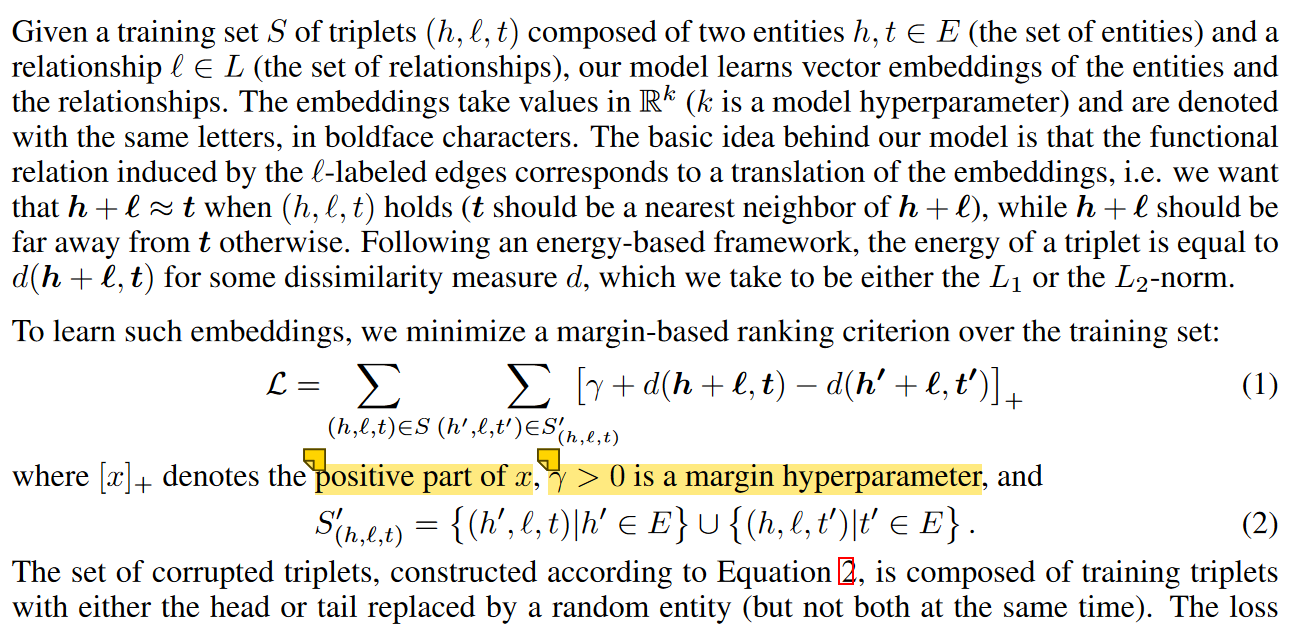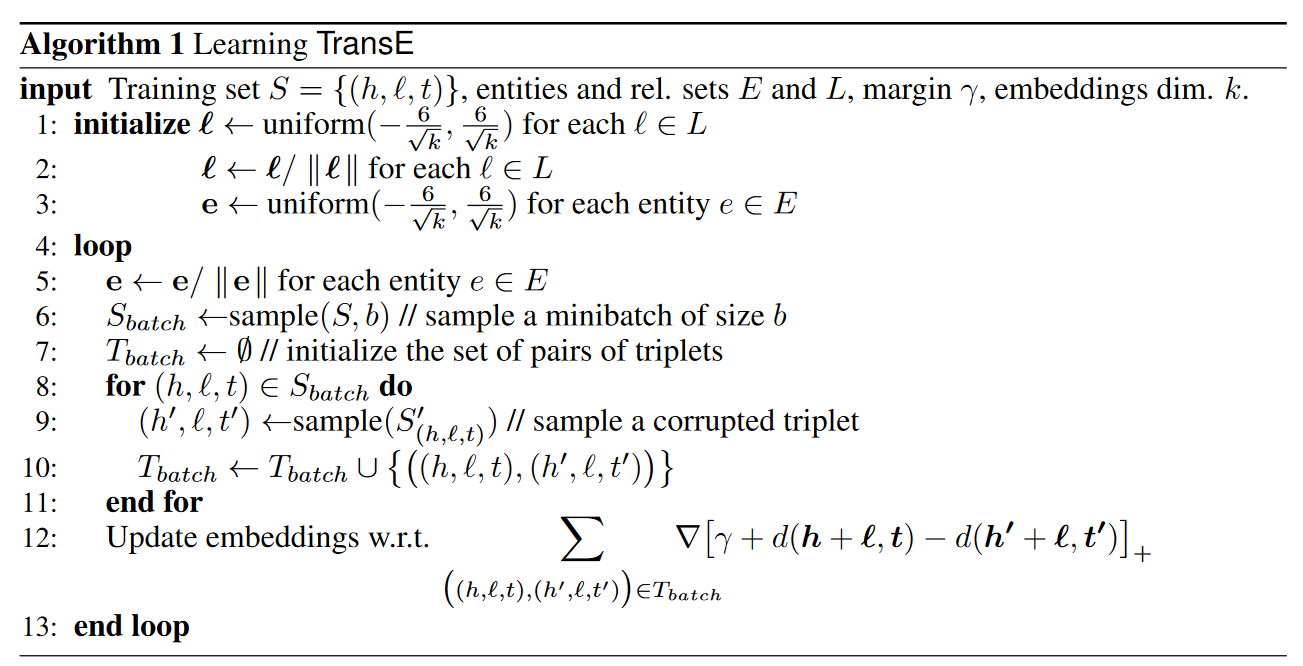## 2. A Joint Embedding Method for Entity Alignment of Knowledge Bases¶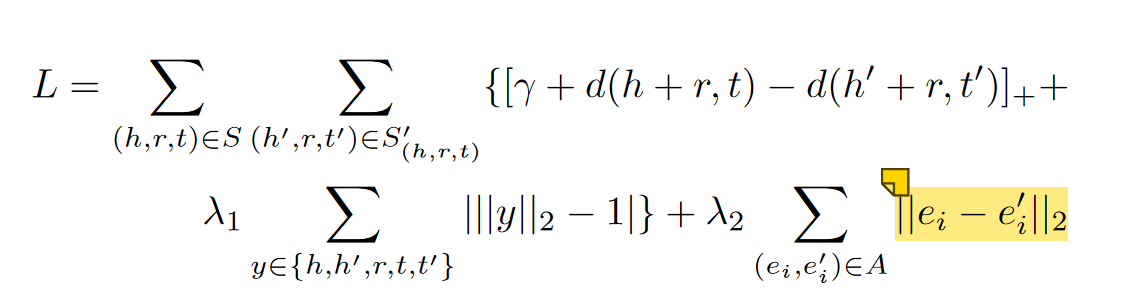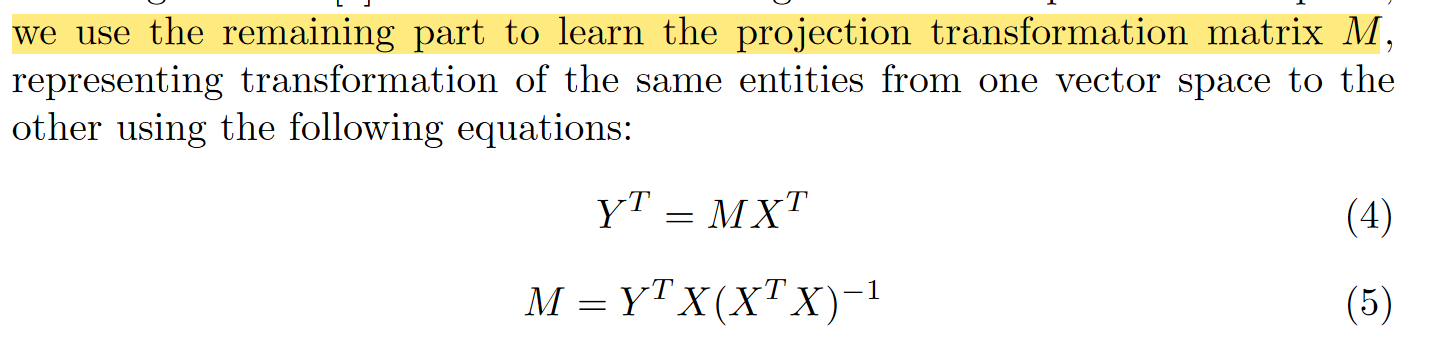## 3. Multilingual Knowledge Graph Embeddings for Cross-lingual Knowledge Alignment¶

• 这样做可以让单语种保留原有特性，因为单个语种的三元组只在自己内部训练
• 不同语言可以并行训练

$S_A=\sum\limits_{T,T'\in\delta(L_i,L_j)}S_a(T,T')$

• 基于距离的坐标轴转换：$S_{a_1}=\mathbf{||h-h'||+||t-t'||}$,$S_{a_2}=\mathbf{||h-h'||+||r-r'||+||t-t'||}$即转换一方的坐标轴使两个对齐
• 转换向量：$S_{a_3}=\mathbf{||h+v^e_{ij}-h'||+||r+v^r_{ij}-r'||+||t+v^e_{ij}-r'||}$
• 线性转换：$S_{a_4}=\mathbf{||M^e_{ij}h-h'||+||M^e_{ij}t-t'||}$,$S_{a_5}=\mathbf{||M^e_{ij}h-h'||+||M^r_{ij}r-r'||+||M^e_{ij}t-t'||}$

## 5. Iterative Entity Alignment via Joint Knowledge Embeddings¶

$L(h,r,t)=\sum\limits_{(h',r',t')\in T^-}[\gamma+E(h,r,t)-E(h',r',t')]_+$

$L(p,r)=\sum\limits_{(h,r',t)\in T^-}[\gamma+E(p,r)-E(p,r')]_+$

Modeling Relation Paths for Representation Learning of Knowledge Bases

$m$通过$r_i$的直接前置，$S_{i}(n,\cdot)$是$S_{i-1}$中任意$n$通过$r_i$的直接后置，$R_p(n)$是从实体$n$获取到的资源

$R_p(h)=1,R_p(h,t)=R_p(t)$

• 转换模型：$E(e_1,e_2)=||\mathbf{e}_1+\mathbf{r}^{(E_1\rightarrow E_2)}-\mathbf{e}_2||$
• 线性变换模型：$E(e_1,e_2)=||\mathbf{M}^{(E_1\rightarrow E_2)}\mathbf{e}_1-\mathbf{e}_2||$，$\mathbb{L}$作为种子
• 前两种方法的分数函数为$J_{T/L}=\sum\limits_{(e_1,e_2)\in \mathbb{L}}\alpha E(e_1,e_2)$
• 参数共享模型：$e_1\equiv e_2,(e_1,e_2)\in\mathbb{L},J_P=0$

$\mathbb{M}$是不断添加新种子的集合

$H_{(e_1,e_2)}=\sum\limits_{(e_1,r,t)}U(e_2,r,t)+\sum\limits_{(h,r,e_1)}U(h,r,e_2)$

TransE中$U(h,r,t)=L(h,r,t)$，PTransE中$U(h,r,t)=L(h,r,t)+\frac 1 Z\sum\limits_{p\in P(h,t)}R(p|h,t)L(p,r)$

## 6. Bootstrapping Entity Alignment with Knowledge Graph Embedding¶

$x,y$是待对齐的实体，对于$\tau=(h,r,t),f(\tau)=||\mathbf{h+r-t}||$

Learning knowledge embeddings by combining limit-based scoring loss

$T^s_{(x,y)}=\{(y,r,t)|(x,r,t)\in T_1^+\}\cup\{(h,r,y)|(h,r,x)\in T_1^+\}\cup\{(x,r,t)|(y,r,t)\in T_2^+\}\cup\{(h,r,x)|(h,r,y)\in T_2^+\}$

$\Delta^{(t)}_{(x,y,y')}=\pi(y|x;\Theta^{(t)})-\pi(y'|x;\Theta^{(t)})$

$\phi_x$用于描述$x$的概率分布，即x获得所有标记的可能性

$\phi_x(\hat y)=\begin{cases}\mathbf 1,x\ is\ labeled\ as\ \hat y\\\frac 1 {|Y'|},x\ is\ unlabeled\end{cases}$

## 7. Co-training Embeddings of Knowledge Graphs and Entity Descriptions for Cross-lingual Entity Alignment¶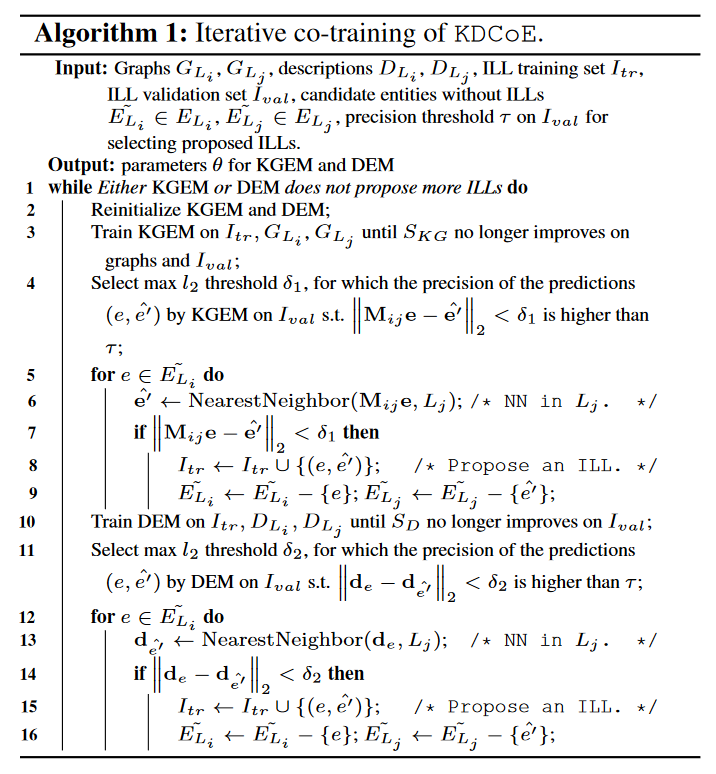ILL：语言间链接

$A(a_{ij})\odot B(b_{ij})=C(c_{ij})$是Hadamard product，$c_{ij}=a_{ij}\times b_{ij}$

《Deep Learning: Adaptive Computation and Machine Learning series》

https://zhuanlan.zhihu.com/p/106276295 ：重置门接近于0时，隐藏层被迫忽略之前的状态，仅用当前输入进行复位，有效地使隐藏状态丢弃将来发现的任何不相关信息；更新门控制将要有多少信息从前一个隐藏状态传递到当前隐藏状态，有助于记住长期信息

An Empirical Exploration of Recurrent Network Architectures

$z_t$是更新门，$g_t$是重置门，$s_t$是状态，$\tilde{s}_t$是对于$x_t$的候选状态

$s_t=\tilde s_t\odot z_t+(1-z_t)\odot s_{t-1}$

$M_z,N_z$是权重矩阵，$b_z$是偏差矩阵

$z_t=\sigma(M_z x_t+N_zs_{t-1}+b_z)$

$M_s,N_s$是权重矩阵，$b_s$是偏差矩阵

$\tilde{s}_t=tanh(M_sx_t+g_t\odot (N_ss_{t-1})+b_s)$

$g_t=\sigma(M_gx_t+N_gs_{t-1}+b_g)$

$u_t=tanh(M_as_t+b_a)$

$a_t=\frac{exp(u^Tx_t)}{\sum\limits_{x_i\in X}exp(u^T_ix_i)}$

$v_t=|X|a_tu_t$

$u_t$是$s_t$的一个隐藏表示，归一化注意力$a_t$是通过softmax计算的，测量$x_t$在对$X$编码中的重要性，应用到$u_t$上获取自注意力输出$v_t$，利用$|X|$包含长度信息

$S_D=\sum\limits_{(e,e')\in \{L_i,L_j\}}-LL_1-LL_2=\sum\limits_{(e,e')\in \{L_i,L_j\}}-log(P(e|e'))-log(p(e'|e))$

$LL_1=log\sigma(d_e^Td_{e'})+\sum\limits_{k=1}^{|B_d|}E_{e_k\sim U(e_k\in E_{L_i})}[log\sigma(-d_{e_k}^Td_{e'})]$

$LL_2=log\sigma(d_e^Td_{e'})+\sum\limits_{k=1}^{|B_d|}E_{e_k\sim U(e_k\in E_{L_j})}[log\sigma(-d_{e}^Td_{e_k})]$

$B_d$是ILL采样，同时负采样另一语言的所有$e_k$，除了$e$

## 8. Non-translational Alignment for Multi-relational Networks¶

TODO: 不全

$\omega_{ij}$是边权重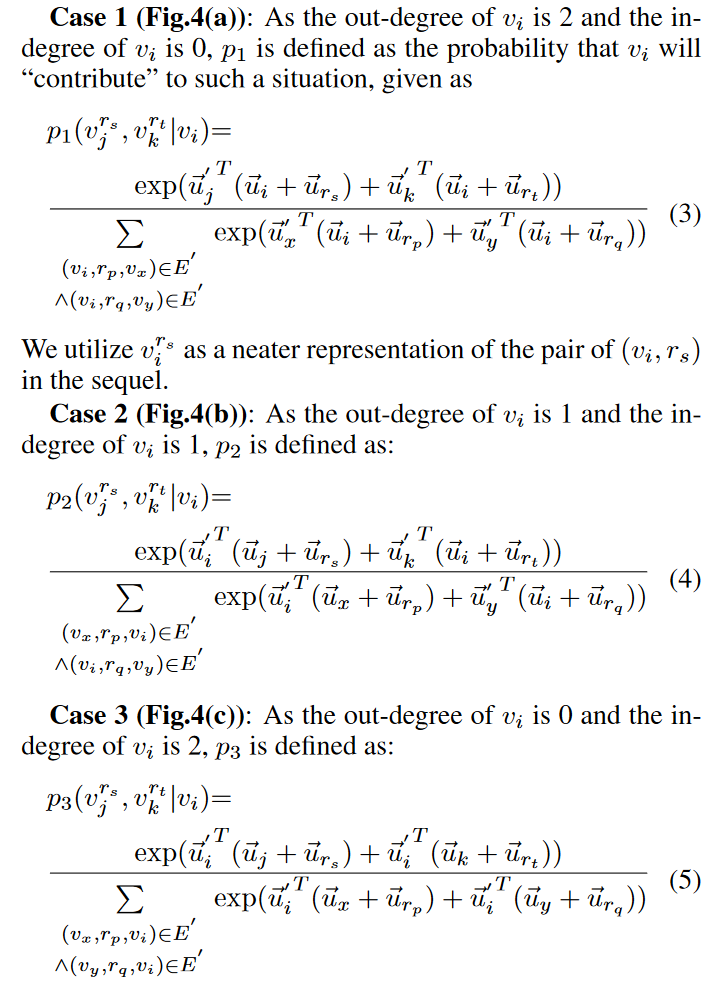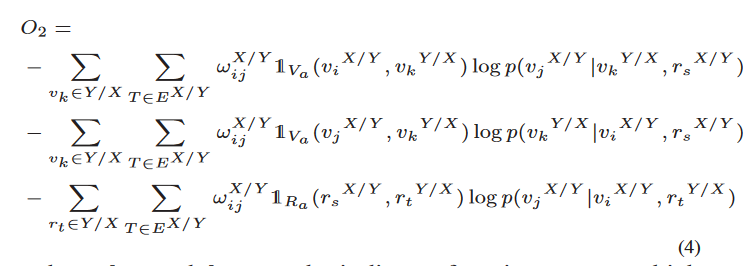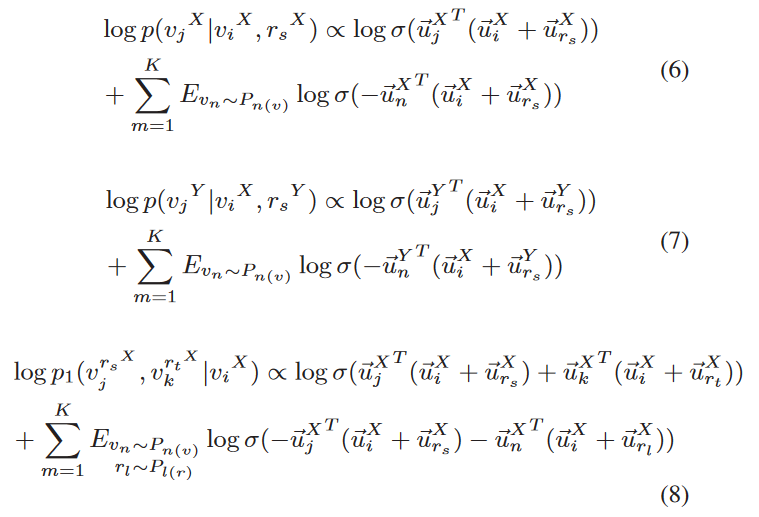• 如果两个实体对齐，则它们的嵌入距离相近
• 如果两个实体对齐，则在一个三元组中替换彼此，得到三元组分数相似

• $c(z)$是聚合后的上下文信息表示，$z$是实体或关系
• $C(z)$是在$z$上下文中出现的部件，$z'$是这些部件的向量表示
• AGG是合并器，可能是Mean,Max,Pooling,LSTM
• 上下文可能有不同影响，因此利用自注意力机制$c(z)=AGG(q(z)*\{z',\forall z'\in C(z)\})$,$q(z)=\frac{exp(\theta_z)}{\sum\limits_{z'\in\{C(z)\}}exp(\theta_{z'})}$,$\theta_z's$是注意力参数
• 实体邻居上下文：$N_c(e)$也是矩阵，对于$t=(e^s,r,e^o)$,$N_c(e^s)$为和$e^s$邻近但是不是$e^o$的实体，使用随机游走获取$k$轮$l$长度范围内的邻居。利用Mean聚合器
• 实体属性上下文：$A_c(e)$，收集$e$的所有实体属性嵌入，利用Max聚合器
• 关系类型上下文：$T_c(r)$，给定一个关系$r$，目标是获取关系中实体类型的影响。通过Mean聚合$r$的类型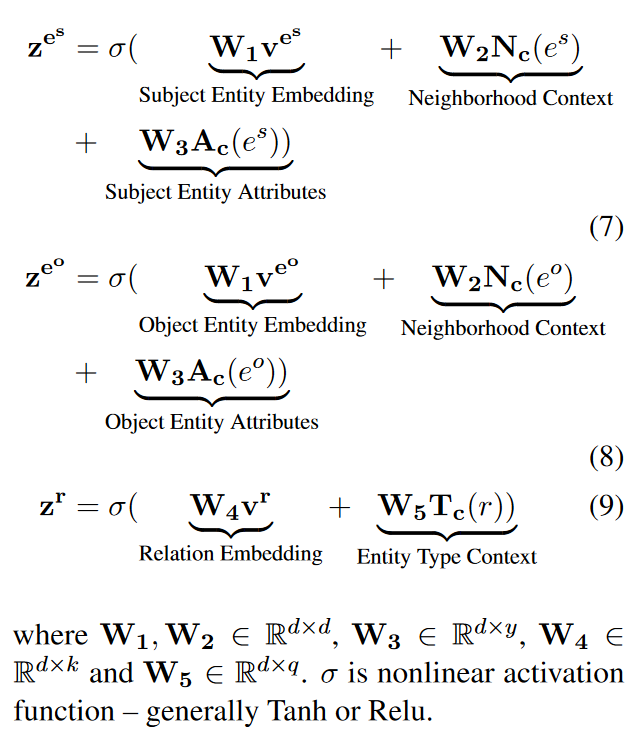## 10. Cross-lingual Knowledge Graph Alignment via Graph Convolutional Networks¶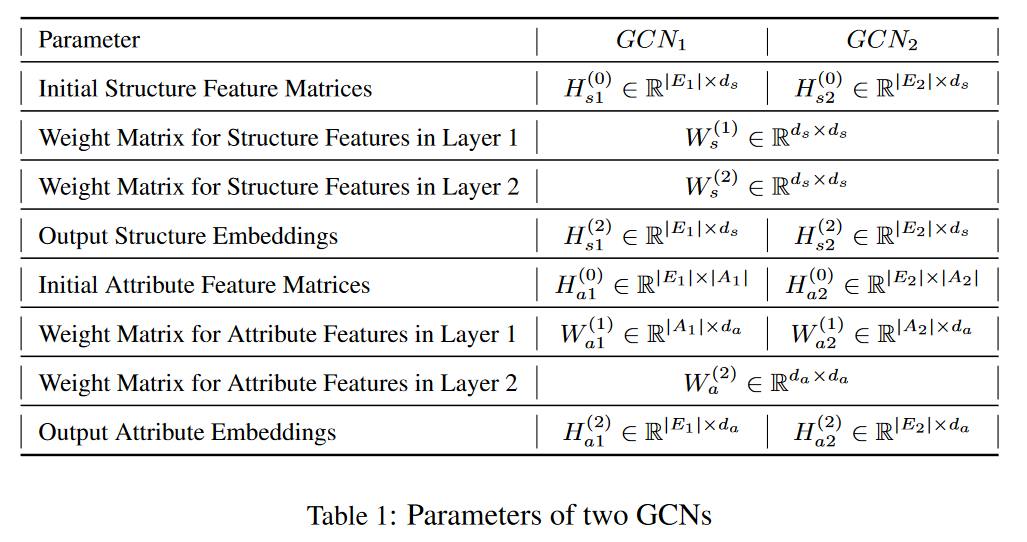$fun(r)=\frac{\#her}{\#tr}$

$ifun(r)=\frac{\#ter}{\#tr}$

$\#her,ter,tr$分别是带有关系$r$的头实体、尾实体和三元组数量

$a_{ij}=\sum\limits_{<e_i,r,e_j>\in G}ifun(r)+\sum\limits_{<e_j,r,e_i>\in G}fun(r)+$

$L_s=\sum\limits_{(e,v)\in S}\sum\limits_{(e',v')\in S'_{(e,v)}}[f(h_s(e),h_s(v))+\gamma_s-f(h_s(e'),h_s(v'))]_+$

$L_s=\sum\limits_{(e,v)\in S}\sum\limits_{(e',v')\in S'_{(e,v)}}[f(h_a(e),h_a(v))+\gamma_a-f(h_a(e'),h_a(v'))]_+$

## Entity Alignment between Knowledge Graphs Using Attribute Embeddings¶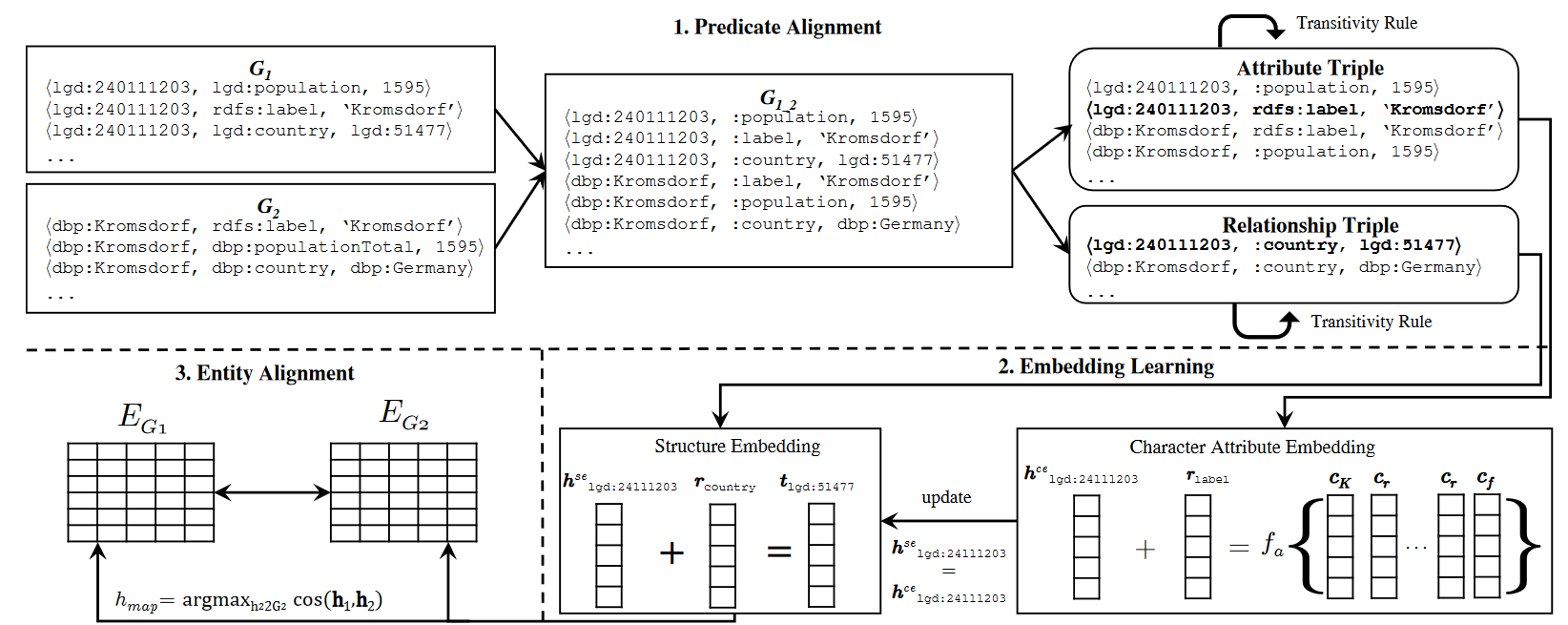$f_a$是组合函数，包括n-gram法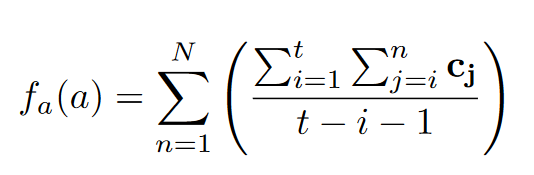和LSTM法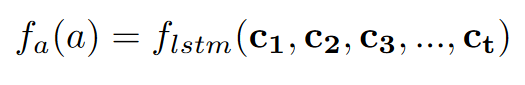## GCN-Align¶

https://zhuanlan.zhihu.com/p/412337460$fun(r)=\frac{\#her}{\#tr}$

$ifun(r)=\frac{\#ter}{\#tr}$

$\#her,ter,tr$分别是带有关系$r$的头实体、尾实体和三元组数量

def func(KG):
cnt = {} # 关系的数量
for tri in KG:
if tri not in cnt:
cnt[tri] = 1
else:
cnt[tri] += 1
r2f = {}
for r in cnt:
return r2f

def ifunc(KG):
tail = {} # 尾实体的数量
cnt = {} # 关系的数量
for tri in KG:
if tri not in cnt:
cnt[tri] = 1
tail[tri] = set([tri])
else:
cnt[tri] += 1
r2if = {}
for r in cnt:
r2if[r] = len(tail[r]) / cnt[r]
return r2if

r2f = func(KG) # r->头实体比例
r2if = ifunc(KG) # r->尾实体比例
M = {}
for tri in KG:
if tri == tri:
continue
if (tri, tri) not in M:
M[(tri, tri)] = max(r2if[tri], 0.3)
else:
M[(tri, tri)] += max(r2if[tri], 0.3)
if (tri, tri) not in M:
M[(tri, tri)] = max(r2f[tri], 0.3)
else:
M[(tri, tri)] += max(r2f[tri], 0.3)
row = []
col = []
data = []
for key in M:
row.append(key)
col.append(key)
data.append(M[key])
return sp.coo_matrix((data, (row, col)), shape=(e, e))

# ...
# 下面用于获取\hat A
# 然后进行一个正规化
d_inv_sqrt = np.power(rowsum, -0.5).flatten()
d_inv_sqrt[np.isinf(d_inv_sqrt)] = 0.
d_mat_inv_sqrt = sp.diags(d_inv_sqrt)


$a_{ij}=\sum\limits_{<e_i,r,e_j>\in G}ifun(r)+\sum\limits_{<e_j,r,e_i>\in G}fun(r)+$

$L_s=\sum\limits_{(e,v)\in S}\sum\limits_{(e',v')\in S'_{(e,v)}}[f(h_s(e),h_s(v))+\gamma_s-f(h_s(e'),h_s(v'))]_+$

$L_s=\sum\limits_{(e,v)\in S}\sum\limits_{(e',v')\in S'_{(e,v)}}[f(h_a(e),h_a(v))+\gamma_a-f(h_a(e'),h_a(v'))]_+$

def align_loss(outlayer, ILL, gamma, k):
left = ILL[:, 0] # 源实体
right = ILL[:, 1] # 目标实体
t = len(ILL)
left_x = tf.nn.embedding_lookup(outlayer, left) # 获取left中所有实体的嵌入
right_x = tf.nn.embedding_lookup(outlayer, right) # 获取right中实体的所有嵌入
A = tf.reduce_sum(tf.abs(left_x - right_x), 1) # L1-norm
neg_left = get_placeholder_by_name("neg_left") # 获取负实体 #tf.placeholder(tf.int32, [t * k], "neg_left")
neg_right = get_placeholder_by_name("neg_right") #tf.placeholder(tf.int32, [t * k], "neg_right")
neg_l_x = tf.nn.embedding_lookup(outlayer, neg_left)
neg_r_x = tf.nn.embedding_lookup(outlayer, neg_right)
B = tf.reduce_sum(tf.abs(neg_l_x - neg_r_x), 1)
C = - tf.reshape(B, [t, k])
D = A + gamma
L1 = tf.nn.relu(tf.add(C, tf.reshape(D, [t, 1]))) # 第一对的损失值
neg_left = get_placeholder_by_name("neg2_left")# 选了第二对负实体 #tf.placeholder(tf.int32, [t * k], "neg2_left")
neg_right = get_placeholder_by_name("neg2_right") #tf.placeholder(tf.int32, [t * k], "neg2_right")
neg_l_x = tf.nn.embedding_lookup(outlayer, neg_left)
neg_r_x = tf.nn.embedding_lookup(outlayer, neg_right)
B = tf.reduce_sum(tf.abs(neg_l_x - neg_r_x), 1)
C = - tf.reshape(B, [t, k])
L2 = tf.nn.relu(tf.add(C, tf.reshape(D, [t, 1]))) # 第二对损失函数
return (tf.reduce_sum(L1) + tf.reduce_sum(L2)) / (2.0 * k * t) # 归一化


(A矩阵过于单薄了吧)

### 环境¶

conda create -n gcn-align python==3.6

conda install cudatoolkit==9 cudnn==7.3.1 scipy==1.1.0 networkx==2.2

pip install tensorflow-gpu==1.10.1

## Relation-Aware Entity Alignment for Heterogeneous Knowledge Graphs¶

$u^r_{ij}$的权重$w^r_{ij}$表示两个关系$r_i,r_j$共享的实体有多相似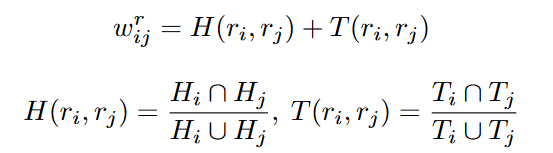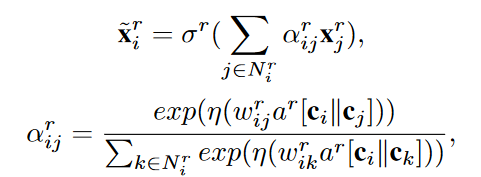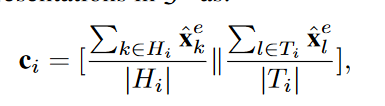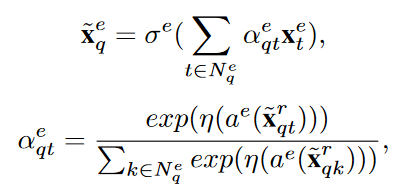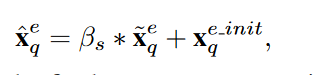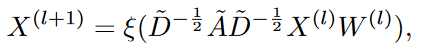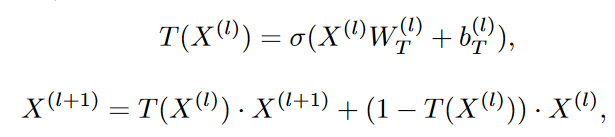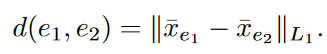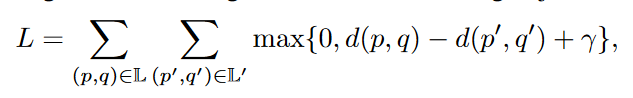## RAGA: Relation-aware Graph Attention Networks for Global Entity Alignment¶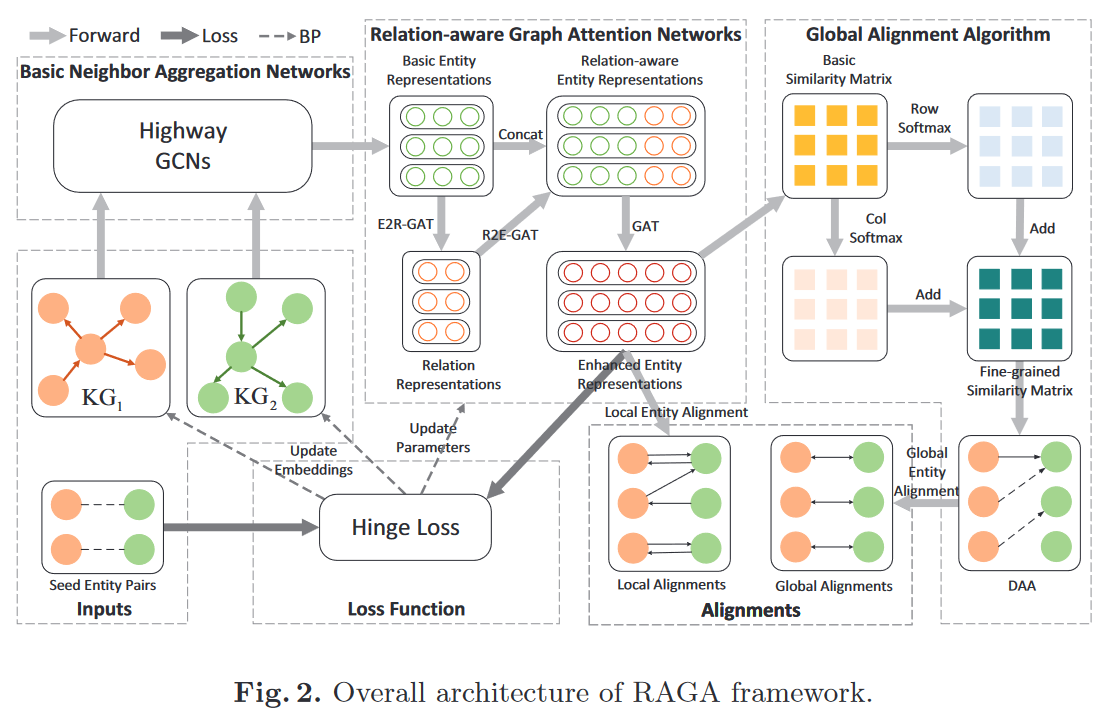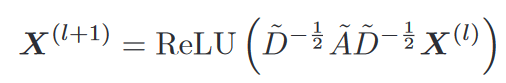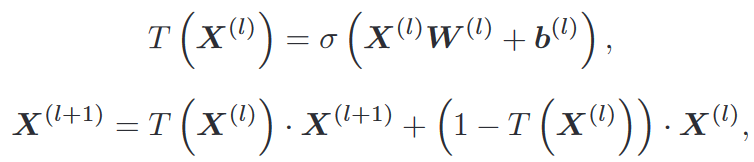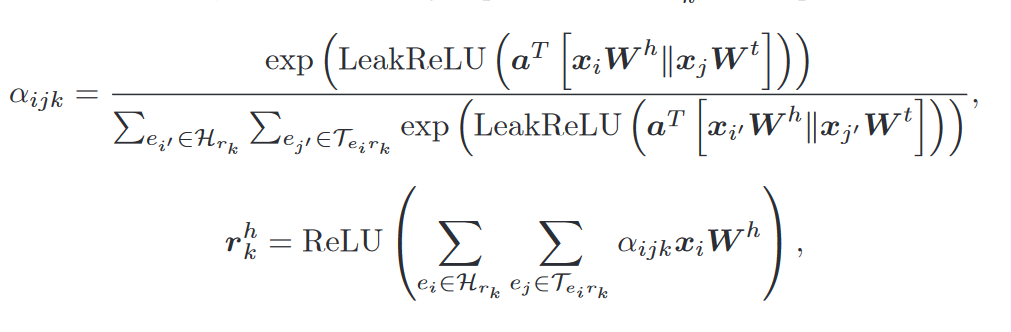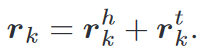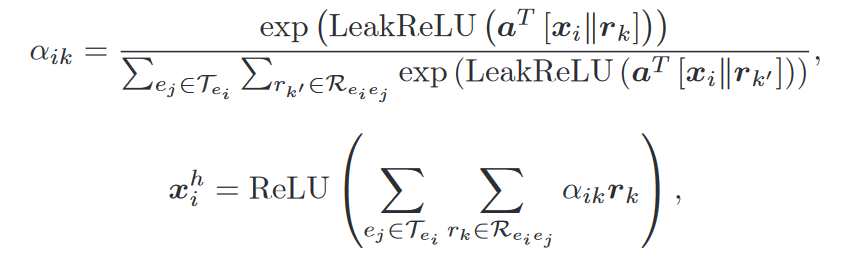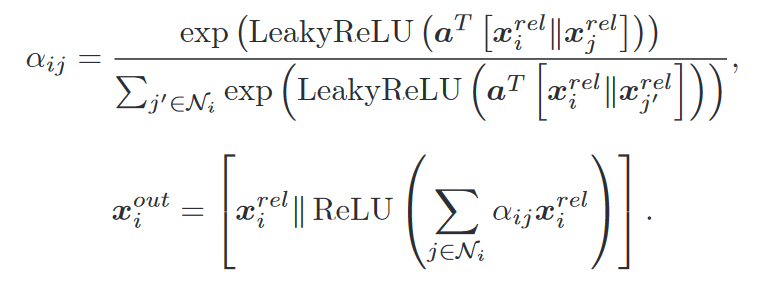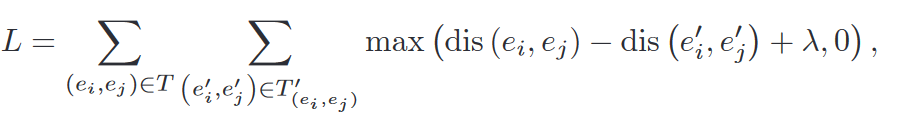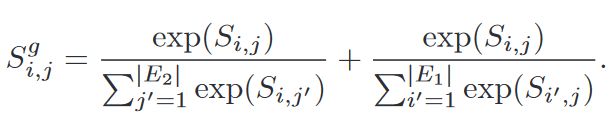### 环境¶

conda install -c "nvidia/label/cuda-11.6.2" cuda-nvprof

clone代码并编译，这里可以提前安装ninja提高编译速度

pip install packaging && git clone https://github.com/NVIDIA/apex.git && cd apex && pip install -v --disable-pip-version-check --no-cache-dir --global-option="--cpp_ext" --global-option="--cuda_ext" ./